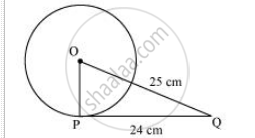Share

# From a point Q, the length of the tangent to a circle is 24 cm and the distance of Q from the centre is 25 cm. The radius of the circle is - CBSE Class 10 - Mathematics

ConceptNumber of Tangents from a Point on a Circle

#### Question

From a point Q, the length of the tangent to a circle is 24 cm and the distance of Q from the centre is 25 cm. The radius of the circle is

• 7 cm

• 12 cm

• 15 cm

• 24.5 cm

#### SolutionLet O be the centre of the circle.

Given that,

OQ = 25cm and PQ = 24 cm

As the radius is perpendicular to the tangent at the point of contact,

Therefore, OP ⊥ PQ

Applying Pythagoras theorem in ΔOPQ, we obtain

OP2 + PQ= OQ2

OP+ 24= 252

OP= 625 − 576

OP= 49

OP = 7

Therefore, the radius of the circle is 7 cm.

Hence, alternative 7 cm is correct.

Is there an error in this question or solution?

#### APPEARS IN

NCERT Solution for Mathematics Textbook for Class 10 (2019 to Current)
Chapter 10: Circles
Ex. 10.20 | Q: 1 | Page no. 213

#### Video TutorialsVIEW ALL 

Solution From a point Q, the length of the tangent to a circle is 24 cm and the distance of Q from the centre is 25 cm. The radius of the circle is Concept: Number of Tangents from a Point on a Circle.
S